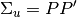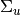# statsmodels.tsa.vector_ar.var_model.VARProcess.orth_ma_rep¶

VARProcess.orth_ma_rep(maxn=10, P=None)[source]

Compute Orthogonalized MA coefficient matrices using P matrix such that. P defaults to the Cholesky decomposition ofParameters: maxn : int Number of coefficient matrices to compute P : ndarray (k x k), optional Matrix such that Sigma_u = PP’, defaults to Cholesky descomp coefs : ndarray (maxn x k x k)